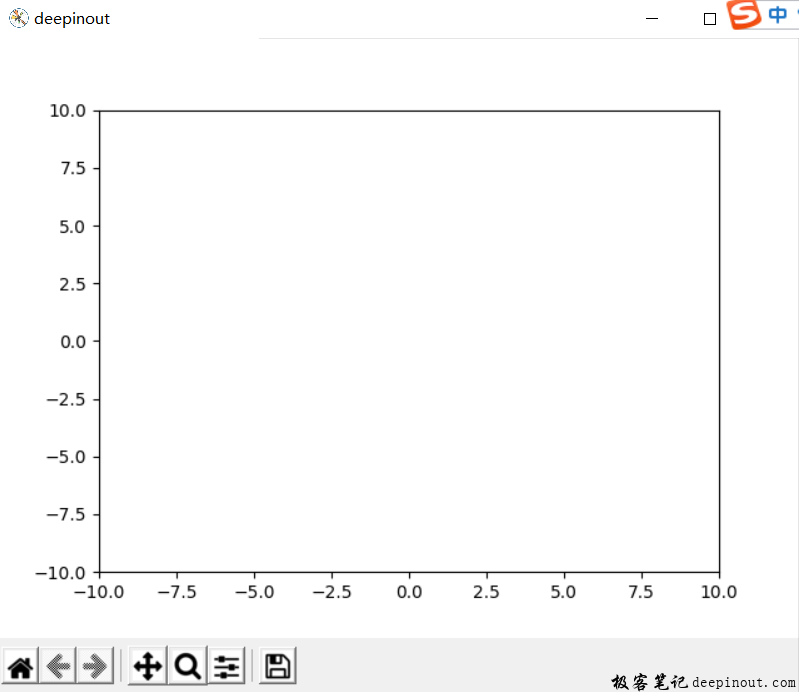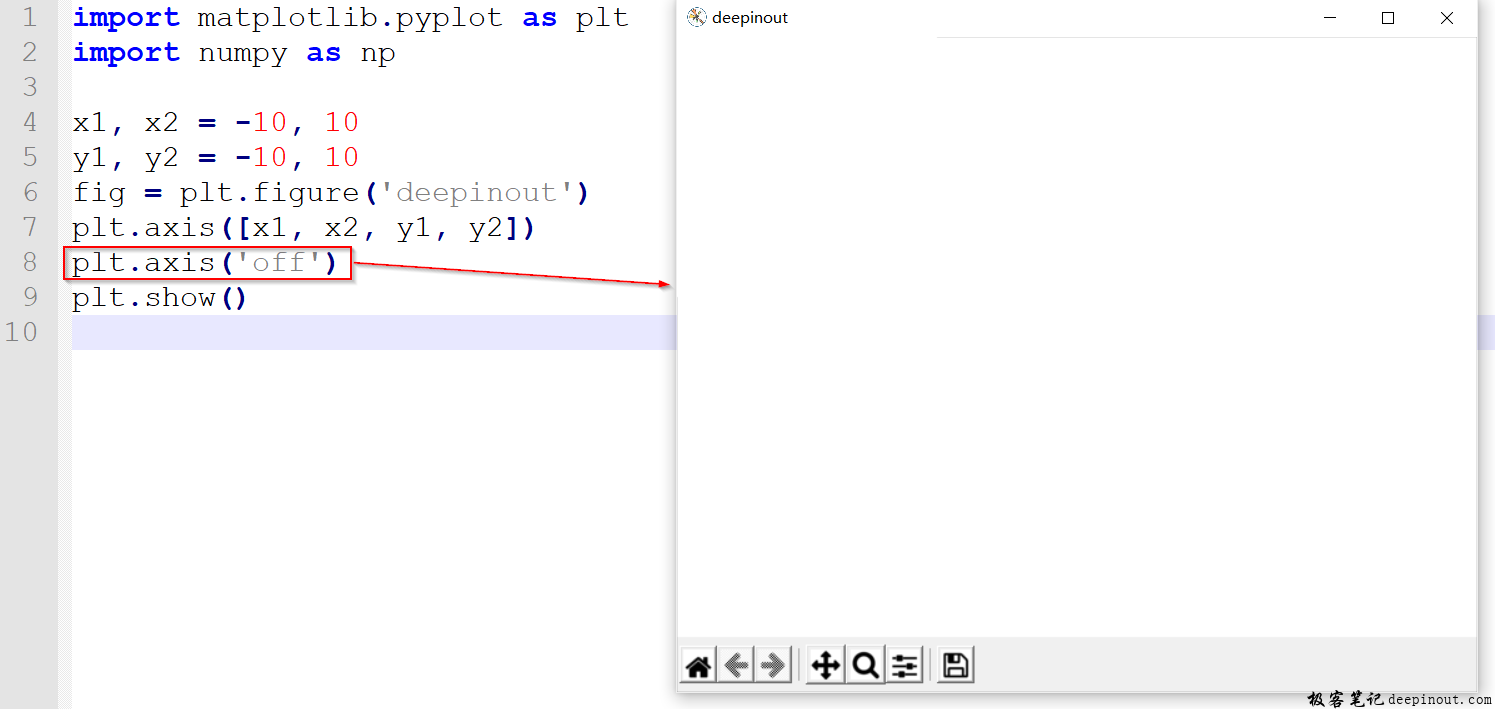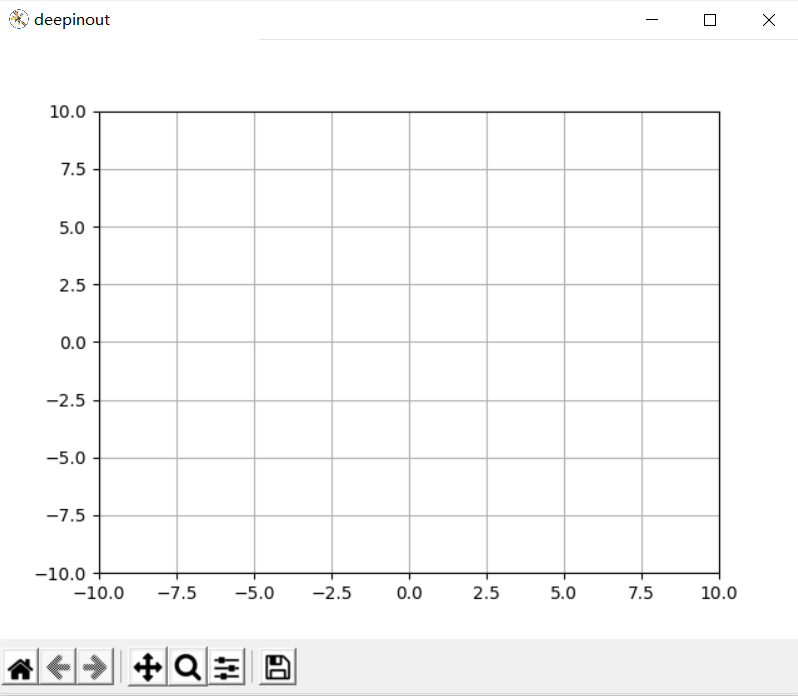# Matplotlib 设置绘图区域大小

Matplotlib 绘图区域学习了怎么样找到区域，也就是找到可以绘画的地方，现在就相当于画家有了笔和纸，这样要考虑怎么样去布局这张白纸了。import matplotlib.pyplot as plt
import numpy as np

x1, x2 = -10, 10
y1, y2 = -10, 10
fig = plt.figure('deepinout')
plt.axis([x1, x2, y1, y2])
plt.show()


plt.axis('on')


import matplotlib.pyplot as plt
import numpy as np

x1, x2 = -10, 10
y1, y2 = -10, 10
fig = plt.figure('deepinout')
plt.axis([x1, x2, y1, y2])
plt.axis('off')
plt.show()import matplotlib.pyplot as plt
import numpy as np

x1, x2 = -10, 10
y1, y2 = -10, 10
fig = plt.figure('deepinout')
plt.axis([x1, x2, y1, y2])
plt.grid(True)
plt.show()• 回顶